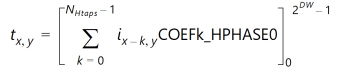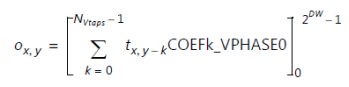# Convert 4:4:4 to 4:2:0 - 2.4 English

## Video Processing Subsystem Product Guide (PG231)

Document ID
PG231
Release Date
2022-10-19
Version
2.4 English

This conversion performs decimation by 2 both vertically and horizontally. This is equivalent to a 2D separable filter implemented by cascading the 4:4:4 to 4:2:2 block and the 4:2:2 to 4:2:0 block. Quantized horizontal filter results are filtered by the vertical filter, which in turn quantizes results back to the [0 .. 2DW-1] range. (DW is the Data Width or number of bits per video component.)

Intermediate 4:2:2 chroma values are computed using This Equation . The resulting computation is shown in This Equation .

Equation 3-16Next, these values are filtered according to This Equation . The resulting computation is shown in This Equation .

Equation 3-17

 X-Ref Target - Figure 3-17The predefined filter coefficients are the same as defined in Convert 4:4:4 to 4:2:2 and Convert 4:2:2 to 4:2:0. In the horizontal direction, decimation is performed with the filter [0.25 0.5 0.25]. The same then happens in the vertical direction.